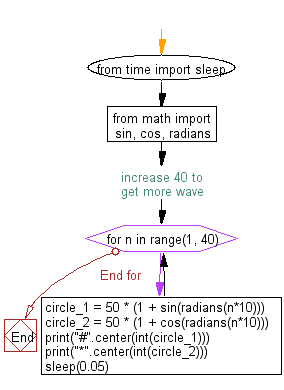﻿ Python Math: Create an ASCII waveform - w3resource# Python Math: Create an ASCII waveform

## Python Math: Exercise-66 with Solution

Write a Python program to create an ASCII waveform.

Sample Solution:-

Python Code:

``````from time import sleep
from math import sin, cos, radians

# increase 40 to get more wave
for n in range(1, 40):
circle_1 = 50 * (1 + sin(radians(n*10)))
circle_2 = 50 * (1 + cos(radians(n*10)))
print("#".center(int(circle_1)))
print("*".center(int(circle_2)))
sleep(0.05)
```
```

Sample Output:

```                            #
*
#
*
#
*
#
*
#
*
#
*
#
*
#
*
#
*
#
*
#
*
#
*
#
*
#
*
#
*
#
*
#
*
#
*
#
*
#
*
#
*
#
*
#
*
#
*
#
*
#
*
#
*
#
*
#
*
#
*
#
*
#
*
#
*
#
*
#
*
#
*
#
*
#
*
#
*

```

Flowchart:## Visualize Python code execution:

The following tool visualize what the computer is doing step-by-step as it executes the said program:

Python Code Editor:

Have another way to solve this solution? Contribute your code (and comments) through Disqus.

What is the difficulty level of this exercise?

Test your Python skills with w3resource's quiz

﻿

## Python: Tips of the Day

Function argument unpacking in Python.

Example:

```def tips_func(x, y, z):
print(x, y, z)

tuple_val = (2, 0, 2)
dict_val = {'x': 3, 'y': 2, 'z': 1}
tips_func(*tuple_val)
tips_func(**dict_val)
```

Output:

```2 0 2
3 2 1
```